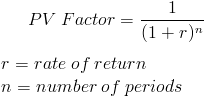# Present Value Factor

PV Factor Calculator (Click Here or Scroll Down)The formula for the present value factor is used to calculate the present value per dollar that is received in the future.

The present value factor formula is based on the concept of time value of money. Time value of money is the idea that an amount received today is worth more than if the same amount was received at a future date. Any amount received today can be invested to earn additional monies.

## Use of the Present Value Factor Formula

By calculating the current value today per dollar received at a future date, the formula for the present value factor could then be used to calculate an amount larger than a dollar. This can be done by multiplying the present value factor by the amount received at a future date.

For example, if an individual is wanting to use the present value factor to calculate today's value of \$500 received in 3 years based on a 10% rate, then the individual could multiply \$500 times the present value factor of 3 years and 10%.

The present value factor is usually found on a table that lists the factors based on the term (n) and the rate (r). Once the present value factor is found based on the term and rate, it can be multiplied by the dollar amount to find the present value. Using the formula on the prior example, the present value factor of 3 years and 10% is .751, so \$500 times .751 equals \$375.66.

## How is the Present Value Factor Formula derived?

The formula for the present value factor is found by breaking down the actual present value formula ofBy factoring out future value, the 2nd portion of the formula is the present value factor which can be used to create a table to simplify the calculation.

New to Finance?